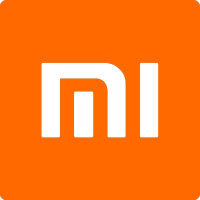# Xiaomi Corp HKEX:1810Xiaomi Corp
HKEX:1810
Price: 15.16 HKD -2.94%
Updated:

## DCF Value

This DCF valuation model was created byAlpha Spread and was last updated on Dec 3, 2023.

Estimated DCF Value of one1810 stock is 15.62 HKD. Compared to the current market price of 15.16 HKD, the stock is Undervalued by 3%.

DCF Valuation FAQ:
DCF Value
Base Case
15.62 HKD
Undervaluation 3%
DCF Value
Price15.62
DCF Value
Xiaomi Corp Competitors:
DCF Valuation
001314
Emdoor Information Co Ltd
300386
Feitian Technologies Co Ltd
002993
Dongguan Aohai Technology Co Ltd
603106
Cashway Fintech Co Ltd
002912
Shenzhen Sinovatio Technology Co Ltd
600100
Tsinghua Tongfang Co Ltd
000977
Inspur Electronic Information Industry Co Ltd
2369

Revenue
277B 430B
Operating Income
12.3B 20.5B
FCFF
10.7B 16.9B

## Present Value Calculation

The first step in the DCF calculation process is to estimate the company's future free cash flow. Once free cash flow is forecasted, it is discounted at a risk-appropriate discount rate. The resulting value is the present value of the company's free cash flow. You can change any model inputs below.

Available Valuation Models
1 valuation model availableUpdated on Dec 3, 2023
Default Valuation
Other investors haven't shared any valuation models for Xiaomi Corp.
Model Settings
Operating Model
Discount Rate
6.85%
Forecast Period
5 Years
Terminal Growth
0%
Discount Rate
6.85%
Terminal Growth
0%
Growth Period
5 Years

### DCF Model Base Case ScenarioDCF Model
Currency: CNY
Billions

To view the process of deriving the DCF Value of one share from the estimated Present Value, see the DCF Value Calculation block.

## DCF Value Calculation

Depending on which type of operating model is chosen (equity or whole firm valuation model), the resulting value is either the value of equity or the value of the entire firm. In the case of the latter, to move from the value of the firm to the value of equity, liabilities are subtracted and assets are added.

### Capital Structure From Present Value to DCF ValuePresent Value 231B CNY + Cash & Equivalents 27.6B CNY + Investments 121B CNY Firm Value 380B CNY - Debt 23B CNY - Minority Interest 265m CNY Equity Value 357B CNY / Shares Outstanding 25B Value per Share 14.27 CNY CNY / HKD Exchange Rate 1.0944 1810 DCF Value 15.62 HKD
Undervalued by 3%

To view the process of calculating the Present Value of Xiaomi Corp' future free cash flow, see the Present Value Calculation block.

Discover More

## What is the DCF value of one 1810 stock?

Estimated DCF Value of one1810 stock is 15.62 HKD. Compared to the current market price of 15.16 HKD, the stock is Undervalued by 3%.

The true DCF Value lies somewhere between the worst-case and best-case scenario values. This is because the future is not predetermined, and the stock's DCF Value is based almost entirely on the future of the company. Knowing the full range of possible stock DCF values gives a complete picture of the investment risks and opportunities.

## How was the DCF Value calculated?

1. Present Value Calculation. Using the DCF Operating Model we projectXiaomi Corp's future free cash flow and discount it at a selected discount rate to calculate its Present Value (231B CNY).

2. DCF Value Calculation. We use the company's capital structure to calculate the total Equity Value based on the previously computed Present Value of the free cash flow. Dividing the Equity Value by the number of shares outstanding gives us the DCF Value of 15.62 HKD per one1810 share.